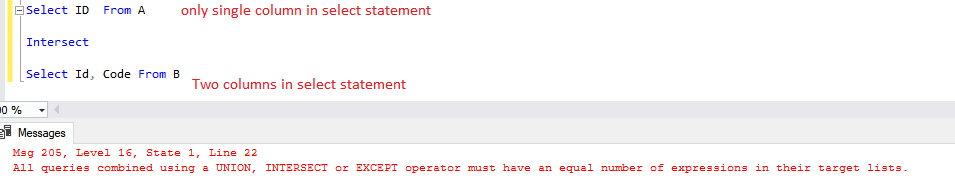Home » SQL SERVER INTERSECT

# SQL SERVER INTERSECT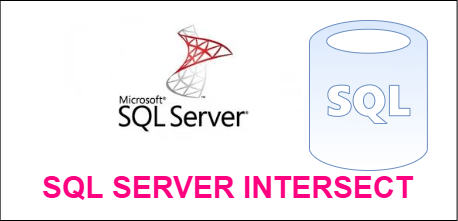The SQL INTERSECT clause is used to combine two SELECT statements, but returns rows only from the first SELECT statement that are identical to a row in the second SELECT statement.

INTERSECT returns only common rows returned by the two SELECT statements.

In Select statement Number of columns and their Data Type must be same. Column Names can be different.

Syntax

```Select col1 , col2 ,.. from tablename where condition

Intersect

select col1 ,col2 ,.. from tablename where condition```

Lets look at an example of INTERSECT in SQL Server.

Firstly, we create two tables and insert some common and different records into them.

```Create table A
( ID int ,
Name Varchar(100) ,
Code Varchar(50)
);

Insert into A Values (1,'Jhon' ,'XYZ001' ) ,
(2,'Mark' ,'ABC002'),
(3,'Alfha' ,'ALF003'),
(4,'Anthony','FGH004') ;

Create table B (
ID int ,
Name Varchar(100) ,
Code Varchar(50
));

Insert into B Values (1,'Jhon' ,'XYZ001' ) ,
(2,'Locus' ,'Loc002'),
(3,'Alfha' ,'ALF003'),
(4,'Jinna','JH004');

```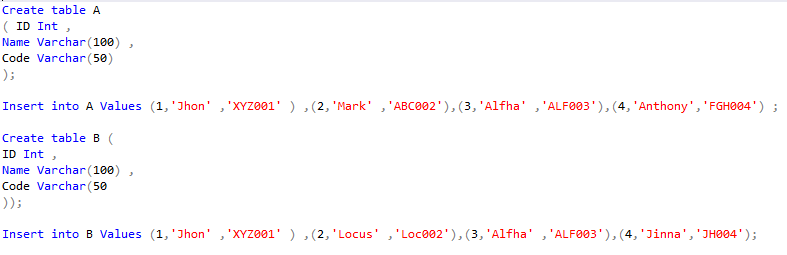Here we have two rows are common in these two table in terms of same values for all columns.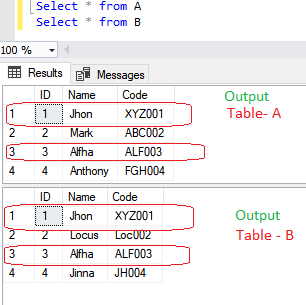Lets apply intersect on table for all columns , It returns the records from first table which are common in second table.

```Select ID, Name, Code From A

Intersect

Select ID, Name, Code From B```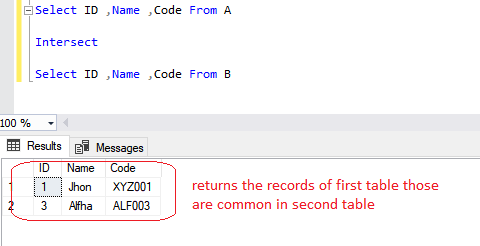Intersect on single column

When you use intersect only on first column of both tables, in this case it returns all the common Ids of first table which are common in second table .

```Select ID  From A

Intersect

Select ID From B```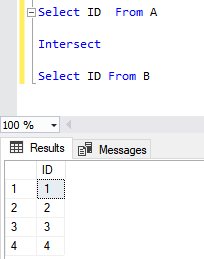#### Error Cases with INTERSECT

Different Column data types  If column data type in select statements for both tables are different , then it returns data type conversions error.

```Select ID From A

Intersect

Select Code From B```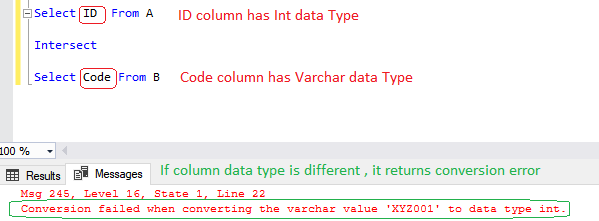Number of Columns in select statement are not equal In this case, it returns an error for not equal expression

```Select ID From A

Intersect

Select ID, Code From B```